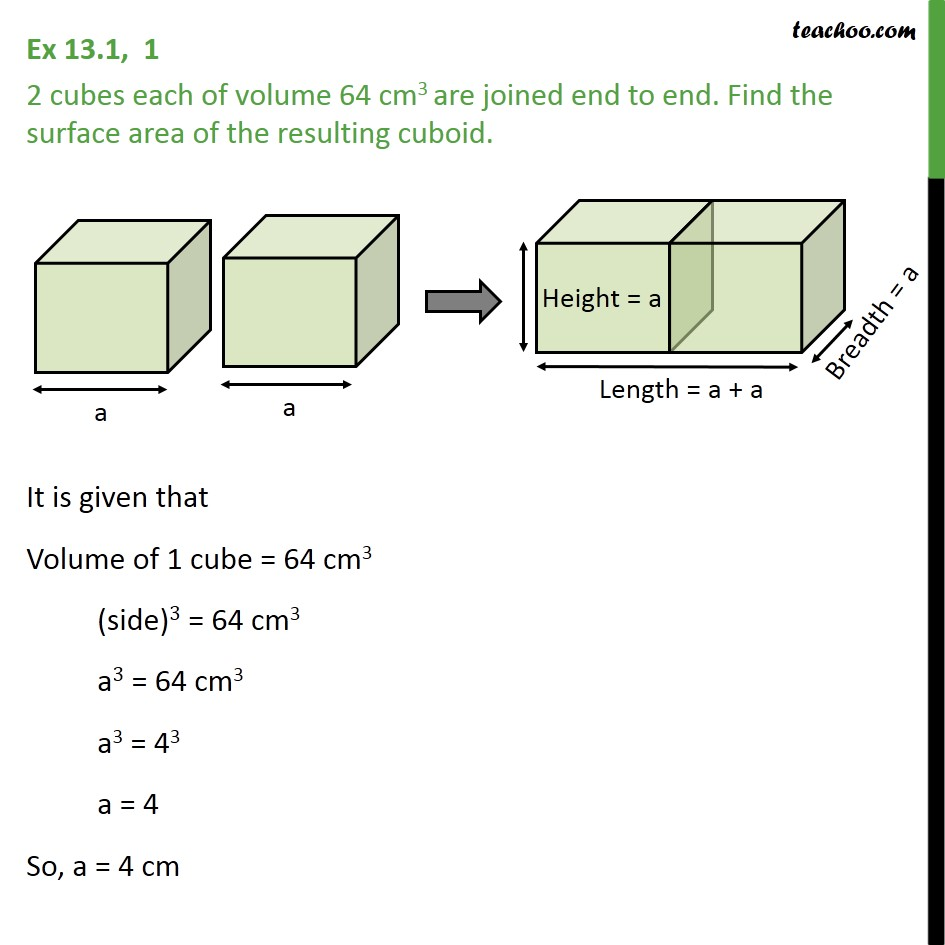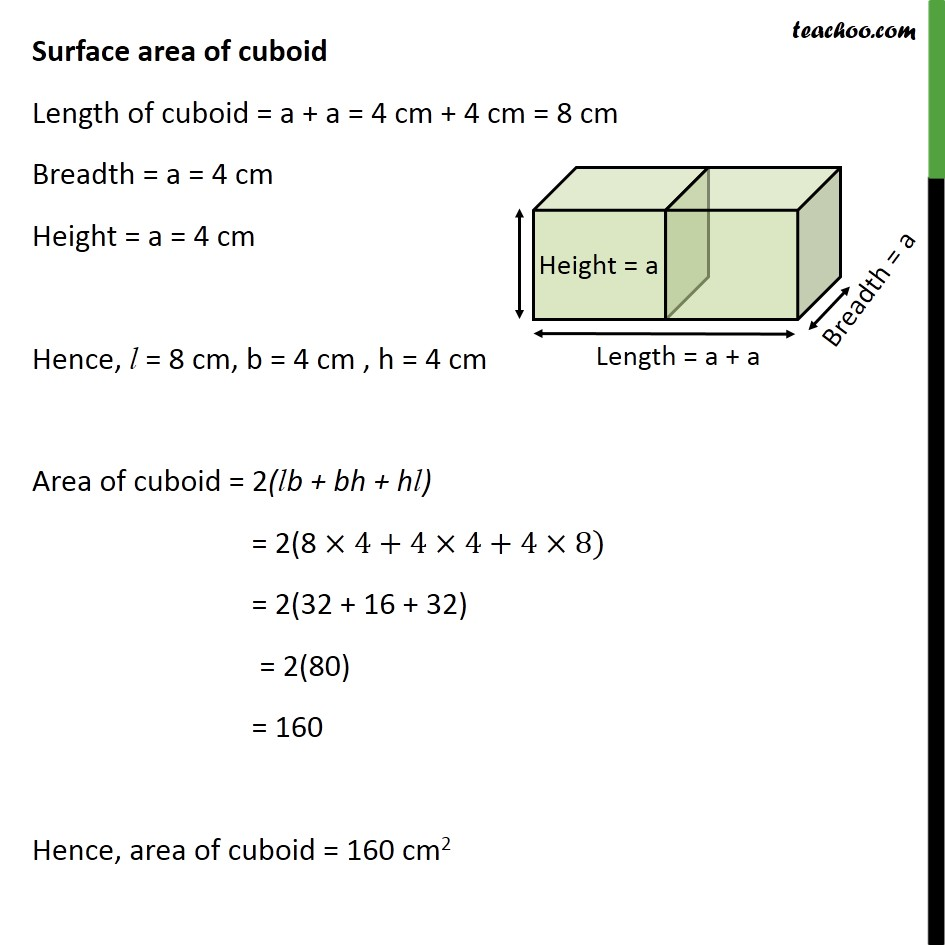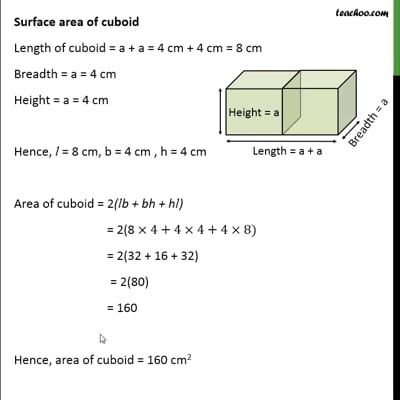Ex 13.1

Chapter 13 Class 10 Surface Areas and Volumes
Serial order wiseThis video is only available for Teachoo black users

Solve all your doubts with Teachoo Black (new monthly pack available now!)

### Transcript

Ex 13.1, 1 2 cubes each of volume 64 cm3 are joined end to end. Find the surface area of the resulting cuboid. It is given that Volume of 1 cube = 64 cm3 (side)3 = 64 cm3 a3 = 64 cm3 a3 = 43 a = 4 So, a = 4 cm Surface area of cuboid Length of cuboid = a + a = 4 cm + 4 cm = 8 cm Breadth = a = 4 cm Height = a = 4 cm Hence, l = 8 cm, b = 4 cm , h = 4 cm Area of cuboid = 2(lb + bh + hl) = 2(8 4+4 4+4 8) = 2(32 + 16 + 32) = 2(80) = 160 Hence, area of cuboid = 160 cm2